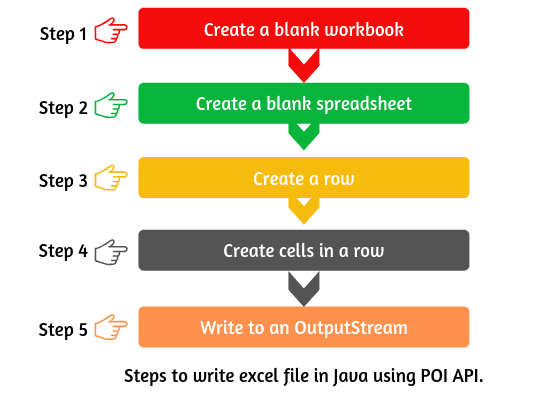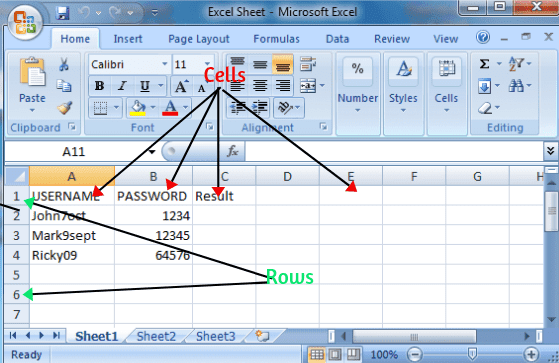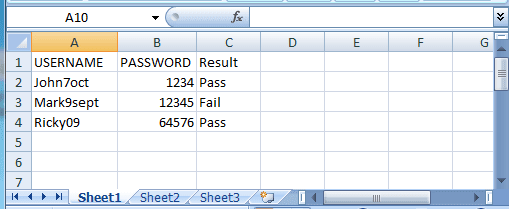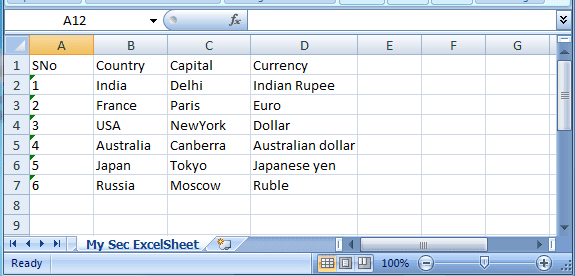# How to Write Excel File in Java using Apache POI

In the previous tutorial, We learned how to read excel file in Java using POI. Now, we will learn how to write excel file in Java using Apache POI in a very simple process and step by step.

## Basic Steps for Writing Excel file in Java using Apache POI

The basic steps for writing data into an excel file using Apache POI API are given below:1. First, create a blank excel sheet (xlsx file) and save it at a particular location. After saving excel file at a particular location, close the created excel file before executing the test script. We have created an Excel file named ExcelSheet and placed it in C drive.

2. Create a blank workbook by using below syntax.
`XSSFWorkbook wb = new XSSFWorkbook();`

3. Create a blank spreadsheet by using below syntax:

`XSSFSheet sheet = wb.createSheet("Sheet Name");`

4. Repeat the following steps a and b until all the data is processed.

a. Create a Row. A spreadsheet consists of rows and cells. It has a grid layout. The rows in the spreadsheet are identified with numbers. Use the following code snippet to create a row in the spreadsheet

`XSSFRow row = sheet.createRow(int arg);`

b. Create cells in a row. A row is a collection of cells. When you enter data in the sheet, it is always stored in the cell. Therefore, you will have to create a cell after creating a row. Use the following syntax to create a cell.

`XSSFCell cell = row.createCell(int columnindex);`

5. Write to an OutputStream. Use the below code to write output stream.

```FileOutputStream fos = new FileOutputStream(filePath);
wb.write(fos);```

6. Close the output stream.

` fos.close();`

Let’s see some example programs to understand the concept of writing the excel file in Java.

Suppose that we have automated a login page of Gmail account with three different usernames and passwords. Data is driven from the excel sheet. After successful log in Gmail page, we have to write the result in the excel sheet using POI API. A sample of excel sheet is showing below.Use the following snippet code for writing the excel sheet.

Program source code 1:

```package excelsheet;
import java.io.File;
import java.io.FileInputStream;
import java.io.FileOutputStream;
import java.io.IOException;
import org.apache.poi.xssf.usermodel.XSSFCell;
import org.apache.poi.xssf.usermodel.XSSFRow;
import org.apache.poi.xssf.usermodel.XSSFSheet;
import org.apache.poi.xssf.usermodel.XSSFWorkbook;

public class WriteExcelSheet
{
public static void main(String[] args) throws IOException
{
// Declare a variable 'filePath' with data type String to store the path of the file.
String filePath = "C:\ExcelSheet\Excel Sheet.xlsx";
// Create an object of the file class and pass the 'filePath' as a parameter to open xlsx based data file.
File file = new File(filePath);
// Create an object of the FileInputStream class and pass the reference variable 'file' as a parameter to read excel data file.
FileInputStream fis = new FileInputStream(file);

// Create the object of XSSFWorkbook class and pass the reference variable 'fis' as a parameter.
XSSFWorkbook wb = new XSSFWorkbook(fis);
// Call getSheet() method to read excel sheet by sheet name.
XSSFSheet sheet=wb.getSheet("Sheet1");
// Call getRow() method to read row by row number.
XSSFRow row = sheet.getRow(1); // Return type of getRow method is a XSSFRow.

// Create a third cell in the second row and set the cell value 'Pass'.
XSSFCell cell = row.createCell(2);
cell.setCellValue("Pass"); // This method returns nothing.
// OR In one line code:
sheet.getRow(1).createCell(2).setCellValue("Pass");

// Similarly, for the third row and third cell.
sheet.getRow(2).createCell(2).setCellValue("Fail");
sheet.getRow(3).createCell(2).setCellValue("Pass");
// Create an object of FileOutputStream class to create the write data in excel file.
FileOutputStream fos = new FileOutputStream(filePath);
// Write data in the excel file.
wb.write(fos);

// Close the output stream.
fos.close();
System.out.println("Result Written Successfully");
}
}```
```Output:
Result Written Successfully.```

Look at the output in the below picture.Let’s take another example program where we have data in the form of an array object. We will write these data in the excel sheet using POI.

Use the following snippet code to write the above data in the excel sheet.

Program source code 2:

```package excelsheet;
import java.io.File;
import java.io.FileInputStream;
import java.io.FileOutputStream;
import java.io.IOException;
import org.apache.poi.ss.usermodel.Cell;
import org.apache.poi.ss.usermodel.Row;
import org.apache.poi.xssf.usermodel.XSSFSheet;
import org.apache.poi.xssf.usermodel.XSSFWorkbook;

public class WriteExcelSheet2
{
public static void main(String[] args) throws IOException
{
// Declare a variable 'filePath' with data type String to store the path of the file.
String filePath = "C:\ExcelSheet\ExcelSheet1.xlsx";
// Create an object of the file class and pass the 'filePath' as a parameter to open xlsx based data file.
File file = new File(filePath);
// Create an object of the FileInputStream class and pass the reference variable 'file' as a parameter to read excel data file.
FileInputStream fis = new FileInputStream(file);
// Create the object of XSSFWorkbook class and pass the reference variable 'fis' as a parameter.
XSSFWorkbook wb = new XSSFWorkbook(fis);

// Create a blank sheet with sheet name 'My First ExcelSheet'.
XSSFSheet sheet = wb.createSheet("My Sec ExcelSheet");
// Create an array object and initialize the data.
Object[][] countryData = {
{"SNo", "Country", "Capital", "Currency"},
{"1", "India", "Delhi", "Indian Rupee"},
{"2", "France", "Paris", "Euro"},
{"3", "USA", "NewYork", "Dollar"},
{"4", "Australia", "Canberra", "Australian dollar"},
{"5", "Japan", "Tokyo", "Japanese yen"},
{"6", "Russia", "Moscow", "Ruble"}
};
// Create rows and iterate them.
int rowCount = 0;
for (Object[] arrayData : countryData)
{
Row row = sheet.createRow(rowCount++);
// Create cells in each row, iterate them and set the cell value.
int columnCount = 0;
for (Object obj : arrayData)
{
Cell cell = row.createCell(columnCount++);
if (obj instanceof String)
{
cell.setCellValue((String)obj);
}
else if (obj instanceof Integer)
{
cell.setCellValue((Integer)obj);
}
}
}
FileOutputStream fos = new FileOutputStream(filePath);
wb.write(fos);
fos.close();
System.out.println("ExcelSheet1.xlsx written successfully");
}
}```
```Output:
ExcelSheet1.xlsx written successfully```

See the output of the excel sheet in the below picture.Let’s take an example program where we will use another technique to write excel file in Java using Apache POI API. Use the following snippet code to write excel file in Java.

Program source code 3:

```package excelsheet;
import java.io.File;
import java.io.FileInputStream;
import java.io.FileOutputStream;
import java.io.IOException;
import java.util.Map;
import java.util.Set;
import java.util.TreeMap;
import org.apache.poi.ss.usermodel.Cell;
import org.apache.poi.xssf.usermodel.XSSFRow;
import org.apache.poi.xssf.usermodel.XSSFSheet;
import org.apache.poi.xssf.usermodel.XSSFWorkbook;

public class WriteExcelSheet3
{
public static void main(String[] args) throws IOException
{
// Declare a variable 'filePath' with data type String to store the path of the file.
String filePath = "C:\ExcelSheet\ExcelSheet1.xlsx";
// Create an object of the file class and pass the 'filePath' as a parameter to open xlsx based data file.
File file = new File(filePath);
// Create an object of the FileInputStream class and pass the reference variable 'file' as a parameter to read excel data file.
FileInputStream fis = new FileInputStream(file);
// Create the object of XSSFWorkbook class and pass the reference variable 'fis' as a parameter.
XSSFWorkbook wb = new XSSFWorkbook(fis);

// Create a blank sheet with sheet name 'My First ExcelSheet'.
XSSFSheet sheet = wb.createSheet("My First ExcelSheet");
// Create an object of Row.
XSSFRow nRow;
// Create an array object and initialize the data.
Object[] obj1 = new Object[]
{ "Name", "Subject", "Highest Marks" };

Object[] obj2 = new Object[]
{ "Deep", "Physics", "98" };

Object[] obj3 = new Object[]
{ "John", "Chemistry", "95" };

Object[] obj4 = new Object[]
{ "Mark", "Maths", "100" };

Object[] obj5 = new Object[]
{ "Shubh", "English", "85" };

Object[] obj6 = new Object[]
{ "Ricky", "Computer Science", "97" }; /

// Create a Map object.
Map < String, Object[] > map = new TreeMap ();
// Call put(Object key, Object value) method to add the entry in the map.
map.put( "1", obj1);
map.put( "2", obj2);
map.put( "3", obj3);
map.put( "4", obj4);
map.put( "5", obj5);
map.put( "6", obj6);

// Iterate over data and write to the sheet.
// Call keySet() method to get set view of all keys.
Set < String > key = map.keySet(); // Return type of keySet method is a Set.

// Declare a variable row with initialization null.
int row = 0;
// Iterating rows using Enhanced for loop.
for (String str : key)
{
// Create row in the sheet and increment by 1.
nRow = sheet.createRow(row++);
// Call get() method to get all the rows. Since they will return an array representation of Objects. Therefore, we will store using a variable 'objArray' with type Object[].
Object [] objArray = map.get(str);

// Since Rows consist of the number of cells. Therefore, we will iterate all cells in each row.
int cell = 0;
// Iterating cells using Enhanced for loop.
for (Object obj : objArray)
{
// Create cells in the rows.
Cell nCell = nRow.createCell(cell++);
nCell.setCellValue((String)obj);
}
}
// Write the workbook in the file system.
FileOutputStream fos = new FileOutputStream(filePath);
wb.write(fos);
fos.close();
System.out.println("ExcelSheet1.xlsx written successfully");
}
}```
```Output:
ExcelSheet1.xlsx written successfully```

See the below output screenshot.Final words
Hope that this tutorial has covered almost all important steps related to how to write excel file in Java using Apache POI. All the steps are very important and keep in mind. If you practice all three programs, we are sure that you will not have any problem to write the excel sheet in Java.